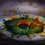# General Knowledge about Primes....

$\quad \quad$ Here's some information that you'll surely like to know about $\textbf{" prime numbers "}$

$\bullet \quad$ We call a positive integer $p$ to be a $\color{#20A900}{\textbf{prime number}}$ if out of the set of all the positive integers, $\color{#D61F06}{\textbf{only}}$ $1$ and $p$ are the divisors of $p$.

$\bullet \quad$ We call a pair of $\color{#20A900}{\textbf{prime numbers}}$ $(p_1,p_2)$ to be $\color{#3D99F6}{\textbf{Twin Primes}}$ iff $\color{#69047E}{\left| p_1 - p_2 \right| = 2}$

$\bullet \quad$ The smallest known pair of $\color{#3D99F6}{\textbf{Twin Primes}}$ is $(3,5)$.

$\bullet \quad$ But what's more interesting, is the $\textbf{BIGGEST KNOWN}$ pair of $\color{#3D99F6}{\textbf{Twin Primes}}$ and it is $\Huge{65516468355 \cdot 2^{333333} \pm 1}$

Both of them are $\color{darkred}{100355}$ digits long.

$\bullet \quad$There are $\color{#20A900}{152891}$ pairs of $\color{#3D99F6}{\textbf{Twin Primes}}$ which are less than $\color{#20A900}{3\times 10^7}$ .

$\bullet \quad$There are $\color{#20A900}{\textbf{only 20 pairs}}$ of $\color{#3D99F6}{\textbf{Twin Primes}}$ between $\color{#20A900}{10^{12}}$ and $\color{#20A900}{10^{12}+10000}$.

$\quad$ This shows the scarcity of $\color{#3D99F6}{\textbf{Twin Primes}}$ as the numbers increase.

$\bullet \quad$ The smallest gap between $2$ consecutive prime numbers is $1$ and it is for the pair $(2,3)$.

$\bullet \quad$ The largest (known till now) gap between $2$ consecutive prime numbers is $\color{#69047E}{1442}$, and it is seen just after the prime $\color{#69047E}{804212830686677669}$. (There are 1441 consecutive composite numbers after this prime).

Source of this information:- $\color{#D61F06}{\text{Elementary Number Theory }} , \color{#3D99F6}{\text{Author- David M. Burton}}$Note by Aditya Raut
6 years, 11 months ago

This discussion board is a place to discuss our Daily Challenges and the math and science related to those challenges. Explanations are more than just a solution — they should explain the steps and thinking strategies that you used to obtain the solution. Comments should further the discussion of math and science.

When posting on Brilliant:

• Use the emojis to react to an explanation, whether you're congratulating a job well done , or just really confused .
• Ask specific questions about the challenge or the steps in somebody's explanation. Well-posed questions can add a lot to the discussion, but posting "I don't understand!" doesn't help anyone.
• Try to contribute something new to the discussion, whether it is an extension, generalization or other idea related to the challenge.
• Stay on topic — we're all here to learn more about math and science, not to hear about your favorite get-rich-quick scheme or current world events.

MarkdownAppears as
*italics* or _italics_ italics
**bold** or __bold__ bold
- bulleted- list
• bulleted
• list
1. numbered2. list
1. numbered
2. list
Note: you must add a full line of space before and after lists for them to show up correctly
paragraph 1paragraph 2

paragraph 1

paragraph 2

[example link](https://brilliant.org)example link
> This is a quote
This is a quote
    # I indented these lines
# 4 spaces, and now they show
# up as a code block.

print "hello world"
# I indented these lines
# 4 spaces, and now they show
# up as a code block.

print "hello world"
MathAppears as
Remember to wrap math in $$ ... $$ or $ ... $ to ensure proper formatting.
2 \times 3 $2 \times 3$
2^{34} $2^{34}$
a_{i-1} $a_{i-1}$
\frac{2}{3} $\frac{2}{3}$
\sqrt{2} $\sqrt{2}$
\sum_{i=1}^3 $\sum_{i=1}^3$
\sin \theta $\sin \theta$
\boxed{123} $\boxed{123}$

## Comments

Sort by:

Top Newest

"Prime numbers" is a big topic and you have included mostly Twin Primes in your note.

I would love to see their special groups and properties even including Mersenne Primes, Weifrich Primes etc. etc. etc. and never ending....

- 6 years, 11 months ago

Log in to reply

Another thing: Recent studies by Yitang Zhang showed that the gaps between primes is at most 70,000,000...

- 6 years, 11 months ago

Log in to reply

@John Ashley Capellan : Your statement is misleading. I can make the gap between two consecutive primes as large as I want. What Yitang Zhang did was prove that there were an infinite number of prime-pairs which differ by less than 70 million.

[$(n+1)!+2, (n+1)!+3, \cdots (n+1)!+n, (n+1)!+(n+1)$ are all composite for any $n\geq 1$. So, prime gaps can be arbitrarily large.]

- 6 years, 11 months ago

Log in to reply

That's great, I will include it... please tell more about it

- 6 years, 11 months ago

Log in to reply

On April 17, 2013, Zhang announced a proof that there are infinitely many pairs of prime numbers which differ by 70 million or less. This proof is the first to establish the existence of a finite bound for prime gaps, resolving a weak form of the twin prime conjecture. Zhang's paper was accepted by Annals of Mathematics in early May 2013. If P(N) stands for the proposition that there is an infinitude of pairs of prime numbers (not necessarily consecutive primes) that differ by exactly N, then Zhang's result is equivalent to the statement that there exists at least one even integer k < 70,000,000 such that P(k) is true. The classical form of the twin prime conjecture is equivalent to P(2); and in fact it has been conjectured that P(k) for all even integers k. While these stronger conjectures remain unproven, a recent result due to James Maynard, employing a different technique, has shown that P(k) for some k ≤ 600. See this Article for more.

- 6 years, 11 months ago

Log in to reply

AWESOME ! Thanks for telling... $\color{darkred}{T}\color{#D61F06}{H}\color{#EC7300}{A}\color{limegreen}{N}\color{#20A900}{K}$ $\color{#3D99F6}{Y}\color{#3D99F6}{O}\color{#69047E}{U}\color{#BA33D6}{!!!}$

- 6 years, 11 months ago

Log in to reply

I think the gap has been lowered to 600

- 6 years, 11 months ago

Log in to reply

×

Problem Loading...

Note Loading...

Set Loading...